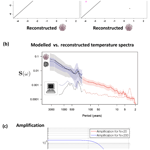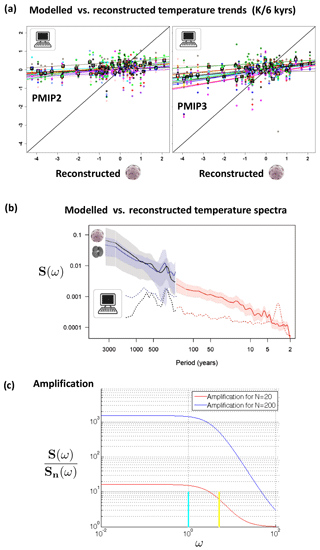https://doi.org/10.5194/esd-9-1279-2018
Special issue:
https://doi.org/10.5194/esd-9-1279-2018# ESD Ideas: The stochastic climate model shows that underestimated Holocene trends and variability represent two sides of the same coin

Gerrit Lohmann
Abstract

Holocene sea surface temperature trends and variability are underestimated in models compared to paleoclimate data. The idea is presented that the local trends and variability are related, which is elaborated in a conceptual framework of the stochastic climate model. The relation is a consequence of the fluctuation–dissipation theorem, connecting the linear response of a system to its statistical fluctuations. Consequently, the spectrum can be used to estimate the timescale-dependent climate response. The non-normality in the propagation operator introduces enhanced long-term variability related to nonequilibrium and/or Earth system sensitivity. The simple model can guide us to analyze comprehensive models' behavior.

Share
Dates

Climate and Earth system models are widely used to evaluate the impact of anthropogenic emissions on future climate. The validation of these models by simulating different climate scenarios is essential to understand the sensitivity of the climate system to external forcing. The models are clearly unrivalled in their ability to simulate a broad range of large-scale phenomena on seasonal to decadal timescales (Flato et al., 2013). However, the reliability of models to simulate climate variability on multidecadal and longer timescales requires additional evaluation. Climate records derived from paleoenvironmental proxy parameters facilitate the testing of models across these timescales.

Interglacial periods provide the means for evaluating the performance of general circulation models in representing sea surface temperature (SST) anomalies and trends (e.g., Lohmann et al., 2013). One key finding is that the models do not capture the magnitude of the derived SSTs from marine proxy records in all climate simulations of the Holocene in which the simulated SST trends systematically underestimate the local marine proxy-based temperature (alkenone) trends. It is suggested that a part of such discrepancies can be caused by either too simplistic interpretation of the proxy data and/or underestimated regional responses in climate models. Figure 1a shows the scatter plot of simulated and reconstructed SST trends for the mid-Holocene to late Holocene, based on results obtained within the Paleoclimate Modelling Intercomparison Project PMIP 2/3 (Braconnot et al., 2007, 2012). Note that the orbital forcing has different signs at high and low latitudes (Berger, 1978). The slopes in Fig. 1a indicate that the response in the models is underestimated by an order of magnitude compared to the SST reconstructions.

By using long-term multi-millennial climate model runs and paleoclimate data, a discrepancy is also detected with respect to variability (Fig. 1b) (see Laepple and Huybers, 2014a, b). While most state-of-the-art climate models realistically simulate interannual variability (in this particular model the interannual variability is overestimated), they underestimate variability on multidecadal to millennial timescales. This was revealed by a systematic comparison of climate model simulations, instrumental records and paleo-observations.Figure 1(a) Global alkenone-based SST trends compared to simulated annual mean SST anomalies in the models listed in PMIP 2 and PMIP 3. The black squares represent the ensemble median mean and the colors correspond to a specific model (see Lohmann et al., 2013). Units are kelvin per 6 kyr. (b) Spectrum of long-term multi-millennial climate model runs and instrumental and paleoclimate data (courtesy of Thomas Laepple, modified from Laepple and Huybers, 2014b). Inherent uncertainties of the paleo-observations are accounted for (compare for instance the blue and black solid lines). (c) Amplification of the spectrum S(ω)∕Sn(ω) of the non-normal two-dimensional dynamics. The amplification can be several orders of magnitude depending on the degree of non-normality related here as the parameter N in Eq. (7).

In order to reconcile both local sensitivity and variability, a model is presented that takes into account the mean as well as the variability, based on Hasselmann (1976). Imagine that the temperature of the ocean is governed by

$\begin{array}{}\text{(1)}& \frac{\mathrm{d}T}{\mathrm{d}t}=-\mathit{\lambda }T+{Q}_{\mathrm{net}}+f\left(t\right),\end{array}$

where the air–sea fluxes due to weather systems are represented by a white-noise process Qnet with zero average $<{Q}_{\mathrm{net}}>=\mathrm{0}$ and δ correlated in time

$\begin{array}{}\text{(2)}& <{Q}_{\mathrm{net}}\left(t\right){Q}_{\mathrm{net}}\left(t+\mathit{\tau }\right)>=\mathit{\delta }\left(\mathit{\tau }\right).\end{array}$

The brackets $<\mathrm{\dots }>$ denote the ensemble mean. The function f(t) is a time-dependent deterministic forcing. We assume furthermore that $f\left(t\right)=c\cdot u\left(t\right)$ with u(t) as a unit step or the so-called Heaviside step function. Because $<{Q}_{\mathrm{net}}>=\mathrm{0}$, the ensemble mean solution is

$\begin{array}{}\text{(3)}& =T\left(\mathrm{0}\right)\cdot \mathrm{exp}\left(-\mathit{\lambda }t\right)+\frac{c}{\mathit{\lambda }}\left(\mathrm{1}-\mathrm{exp}\left(-\mathit{\lambda }t\right)\right),\end{array}$

where we have $=T\left(\mathrm{0}\right)$. As an equilibrium response we have

$\begin{array}{}\text{(4)}& \mathrm{\Delta }T=\underset{t\to \mathrm{\infty }}{lim}=\frac{c}{\mathit{\lambda }}.\end{array}$

The fluctuations can be characterized by the spectral Fourier component ${\stackrel{\mathrm{^}}{T}}_{\mathit{\omega }}=\frac{{\stackrel{\mathrm{^}}{Q}}_{\mathit{\omega }}}{\left(\mathit{\lambda }+i\mathit{\omega }\right)}$ with the spectrum

$\begin{array}{}\text{(5)}& S\left(\mathit{\omega }\right)=<\stackrel{\mathrm{^}}{T}{\stackrel{\mathrm{^}}{T}}^{*}>=\frac{<\stackrel{\mathrm{^}}{Q}{\stackrel{\mathrm{^}}{Q}}^{*}>}{{\mathit{\lambda }}^{\mathrm{2}}+{\mathit{\omega }}^{\mathrm{2}}}=\frac{\mathrm{1}}{{\mathit{\lambda }}^{\mathrm{2}}+{\mathit{\omega }}^{\mathrm{2}}}\end{array}$

showing that a too high value in λ is related to a too low low-frequency variance. The model–data differences on long timescales suggest that feedback mechanisms and internal variability may not be represented well in current climate models. The relation of Eqs. (4) and (5) is related to the fluctuation–dissipation theorem connecting the linear response to the statistical fluctuations (Nyquist, 1928), and the relation of a too low sensitivity (Fig. 1a) and too low variability (Fig. 1b) is qualitatively detected. Recently, one focus of research was to identify feedback mechanisms in the Earth system enhancing the sensitivity (Stärz et al., 2016) or variability (Bakker et al., 2017).

If we include more components and feedbacks in the system, we can introduce higher values for the climate sensitivity, called Earth system sensitivity. In the spectral domain, we can consider a series of processes like Eq. (1) and the spectrum is the sum

$\begin{array}{}\text{(6)}& {S}_{n}\left(\mathit{\omega }\right)=\sum _{i}\frac{\mathrm{1}}{{\mathit{\lambda }}_{i}^{\mathrm{2}}+{\mathit{\omega }}^{\mathrm{2}}}\end{array}$

over the components in Eq. (5). However, in the fluid dynamical context we typically have a non-normal matrix with non-orthogonal eigenvectors with S(ω)>Sn(ω). The plausible physical interpretation of the nature of the non-diagonal terms is related to the extraction of energy from a mean state and/or mean flow. In terms of the simple Stommel ocean model, this is the mean ocean circulation (Lohmann and Schneider, 1999). In fluid dynamical context, this has been discussed in terms of shear flow instabilities (e.g., Trefethen et al., 1993; Farrell and Ioannou, 1996).

Consider as an example a two-dimensional system with a 2×2 matrix

$\begin{array}{}\text{(7)}& \mathbf{A}=\left[\begin{array}{cc}-\mathrm{1}& N\\ \mathrm{0}& -\mathrm{5}\end{array}\right]\end{array}$

replacing the scalar number λ in Eq. (1) and unit matrix in Eq. (2). The eigenfrequencies are independent of N (yellow and cyan vertical lines in Fig. 1c). However, S(ω) can be amplified relative to Sn(ω) by orders of magnitude (Fig. 1c), affecting the low-frequency climate variability. The spectra in Fig. 1c are calculated analytically. For real problems, the estimation of A can be carried out via the principal oscillation pattern method (Hasselmann, 1988) in which the dynamical propagator has in general a non-normal structure. As a logical next step, the spectral properties can be used to estimate the timescale-dependent climate and Earth system sensitivity.

Data availability

The data sets with their links are documented in Lohmann et al. (2013) and Laepple and Huybers (2014a, b).

Competing interests

The author declares that there is no conflict of interest.

Acknowledgements

The paper is supported by the AWI research program PACES, and the REKLIM, PalMod, and SiGePax projects. Thanks go to Thomas Laepple for panel Fig. 1b.

The article processing charges for this open-access
publication were covered by a Research
Centre of the Helmholtz Association.

Edited by: Anders Levermann
Reviewed by: Michel Crucifix and one anonymous referee